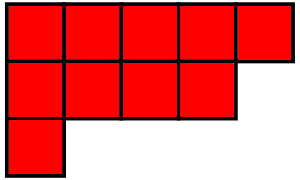### Schur FunctorsThe representation theory of the symmetric groups is clarified by thinking of all representations of all these groups as objects of a single category: the category of Schur functors. These play a universal role in representation theory, since Schur functors act on the category of representations of any group. We can understand this as an example of categorification. A "rig" is a "ring without negatives", and the free rig on one generator is $$\mathbb{N}[x]$$, the rig of polynomials with natural number coefficients. Categorifying the concept of commutative rig we obtain the concept of "symmetric 2-rig", and it turns out the category of Schur functors is the free symmetric 2-rig on one generator. Thus, in a certain sense, Schur functors are the next step after polynomials.

You can see the slides here, and also a video from when I gave the talk at Ohio State: